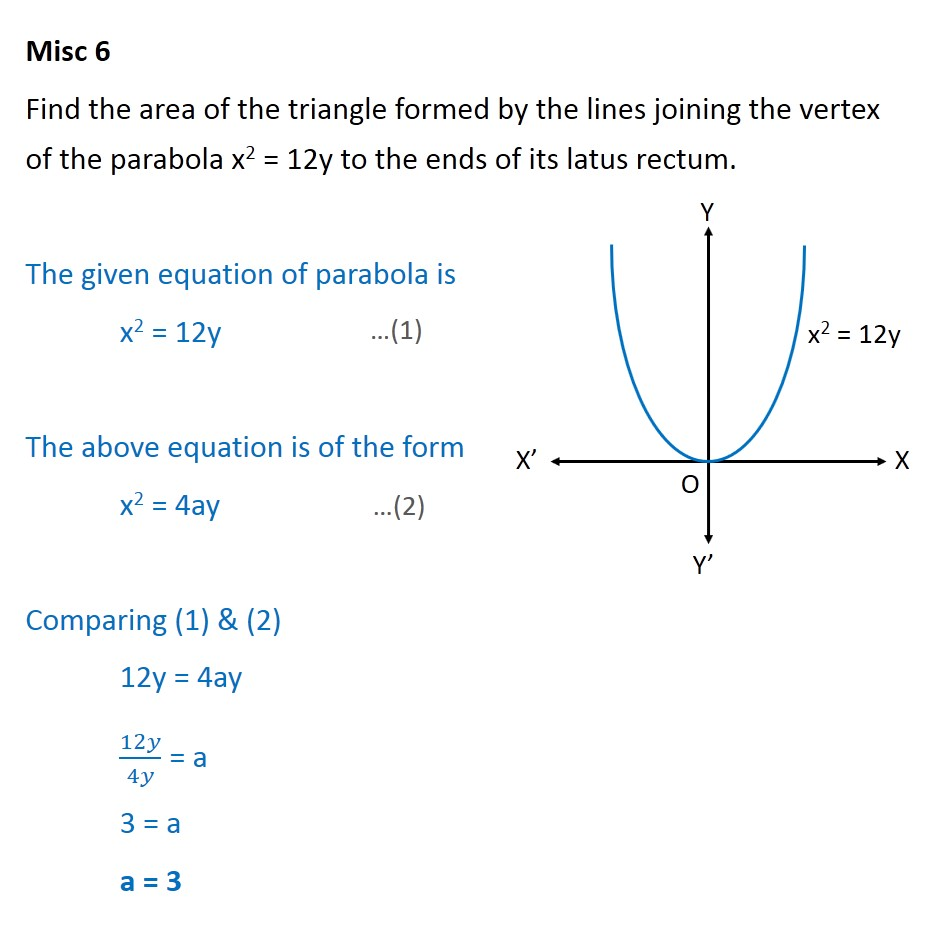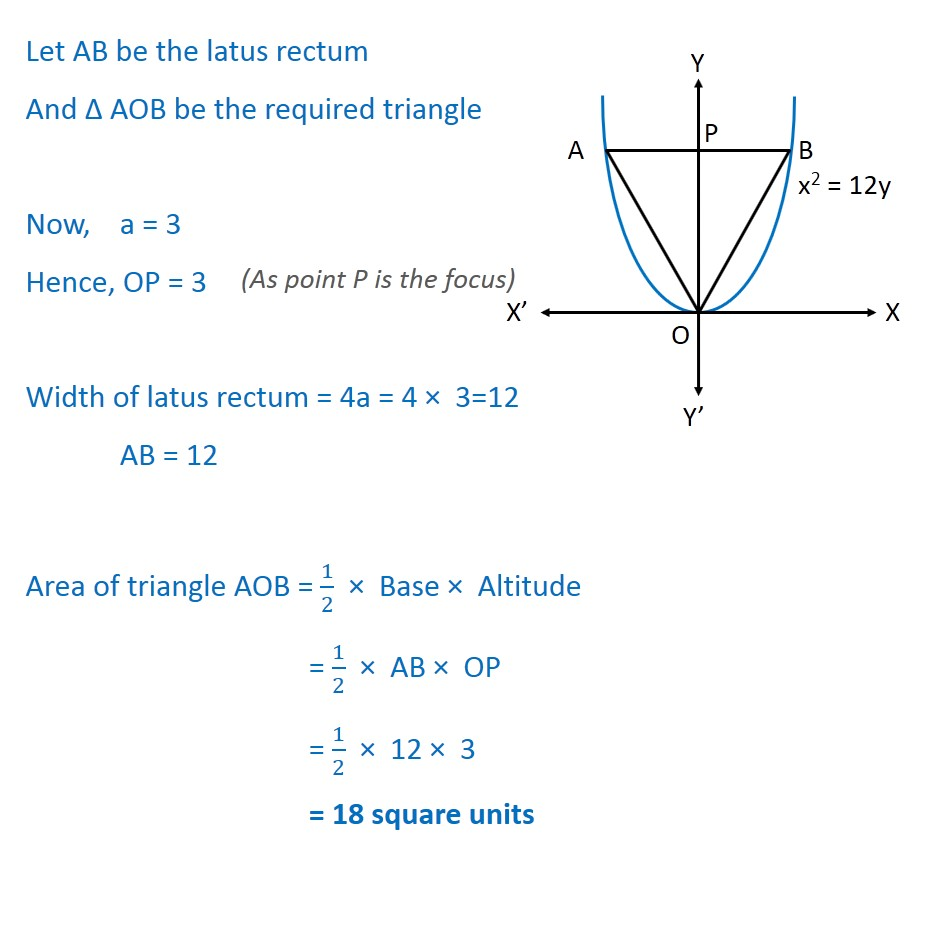Parabola - Triangle in parabola problemLearn in your speed, with individual attention - Teachoo Maths 1-on-1 Class

### Transcript

Misc 6 Find the area of the triangle formed by the lines joining the vertex of the parabola x2 = 12y to the ends of its latus rectum. The given equation of parabola is x2 = 12y The above equation is of the form x2 = 4ay Comparing (1) & (2) 12y = 4ay 12 4 = a 3 = a a = 3 Let AB be the latus rectum And AOB be the required triangle Now, a = 3 Hence, OP = 3 Width of latus rectum = 4a = 4 3=12 AB = 12 Area of triangle AOB = 1 2 Base Altitude = 1 2 AB OP = 1 2 12 3 = 18 square units